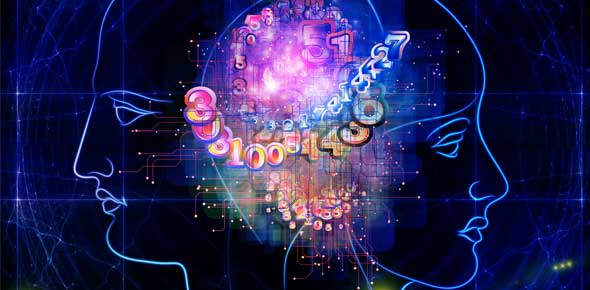# Online Test-2 Reasoning (Banks)

20 Questions | Total Attempts: 129SettingsRelated Topics
• 1.
If  Neha says, ”Amruta’s  father Raj is the only son my      father-in-law Mahesh.” Then how Bindu, who is the sister of      Amruta is related to Mahesh ?
• A.

Daughter

• B.

Wife

• C.

Daughter-in-Law

• D.

Niece

• E.

None

• 2.
Pointing to a gentleman, Deepak said, “His only brother is     the father of my daughter’s father.” How is the gentleman      related to Deepak ?
• A.

Father

• B.

Grandfather

• C.

Brother-in-Law

• D.

Uncle

• E.

None

• 3.
Q’s mother is the sister of R and daughter of  S. N is the    daughter of R and  sister of M. How is M related to S ?
• A.

Son

• B.

Son’s Son

• C.

Brother

• D.

Daughter’s son

• E.

• 4.
• A.

Daughter

• B.

Wife

• C.

Mother

• D.

Grandmother

• E.

None

• 5.
• A.

Niece

• B.

Daughter

• C.

Sister

• D.

Mother

• E.

None of these

• 6.
Pointing towards a boy , Aruna said to Pushpa, “The      mother of his father is the wife of your grandfather       (Mother’s father )”.How  Pushpa is related to that boy ?
• A.

Sister

• B.

Niece

• C.

Cousin sister

• D.

Wife

• E.

None of these

• 7.
The son of  M is the father of N and grandfather       (Mother’s father ) of R. S is the daughter of N snd sister of  B.     On the basis of this information, how is  M related to B ?
• A.

Grandfather

• B.

Grandmother

• C.

• D.

Grandmother’s mother

• E.

Grandfather’s father

• 8.
A is the brother of B, C is the brother of A. To establish a     relationship between B and C, which of the following     information is  required ?   1)Sex of  C                      2) Sex of B
• A.

Only 1 is required

• B.

Only 2 is required

• C.

Both 1 and 2 are required

• D.

Neither 1 Nor 2 is required

• E.

None

• 9.
Introducing a man, Neeraj said , “His wife is the only    daughter of my wife .” How is Neeraj related to that man ?
• A.

Father

• B.

Grandfather

• C.

Father-in-Law

• D.

Son

• E.

Brother

• 10.
Shyama says that father of Rajiv’s father is my father.     How is Shyama related to Rajiv ?
• A.

Sister

• B.

Mother

• C.

Aunt

• D.

Niece

• E.

Mother’s mother

• 11.
Pointing to Raman in the photograph, Aditi  said , “The     only son of his mother is my father . “How is Aditi related     to Raman ?
• A.

Mother’s sister

• B.

Bua

• C.

Daughter

• D.

Niece

• E.

None

• 12.
Pointing towards a lady, a man said , “the father of my       grandfather .”How is the lady related to that man ?
• A.

Sister

• B.

Daughter

• C.

Aunt

• D.

Mother-in-Law

• E.

None

• 13.
A man said to a woman, “The only sister of your brother       is my mother.” How is  the man related to the man ?
• A.

Father

• B.

Son

• C.

Husband

• D.

Brother

• E.

Grandfather

• 14.
Pointing towards a man in the photograph , Archana said ,     “He is the son of  the only of my grandfather .”How  is  the       man related to Archana ?
• A.

Cousin

• B.

Nephew

• C.

Brother

• D.

Son

• E.

None of these

• 15.
Pointing towards a woman in the photograph , Rajesh        said, “The only daughter of  her grandfather is my wife .”       How is Rajesh related to that woman ?
• A.

Uncle

• B.

Father

• C.

Maternal Uncle

• D.

Brother

• E.

None

• 16.
Pointing towards a man in the photograph, a lady said,       “The father of his brother is the only son of my mother.”
• A.

Brother

• B.

Son

• C.

Cousin

• D.

Grandson

• E.

Nephew

• 17.
A+B means ‘ A is the brother of B’, A÷B means ‘ A is the       father of B’ and A X B means ‘A is the sister of B’.       Which of the following means ‘M’ is the uncle of ‘P’ ?
• A.

M÷NXP

• B.

NXP÷M

• C.

M+S÷R+P

• D.

M+K÷TXP

• E.

None

• 18.
‘P+Q’ means ‘ P is the brother of Q’. ‘P-Q’ means ‘p is        the mother of Q’, and ‘PXQ’ means ‘P is the sister of Q’.        Which of the following means that M is the maternal-        uncle of R?
• A.

M-R+K

• B.

M+K-R

• C.

M+KXQ

• D.

There is no such symbol

• E.

None of these

• 19.
If SXT means ‘S is brother of T’, S+T means ‘S is the       father of T’, which of the following shows ‘O’ is the       cousin of R?
• A.

R X T + O

• B.

R + T X O

• C.

R X O X T

• D.

T X O + R

• 20.
If ‘P + Q’ means ‘ P is the sister of Q’, ‘P-Q’ means ‘ P is       the mother of Q’, ‘P X Q’ means ‘P is the brother of Q’,     ‘P÷Q’ means ‘P is the father of Q’.  Which of the following     means M is the maternal-uncle of R?
• A.

MXT-R

• B.

M÷TXR

• C.

M+T÷K-R

• D.

M÷N+J

• E.

None